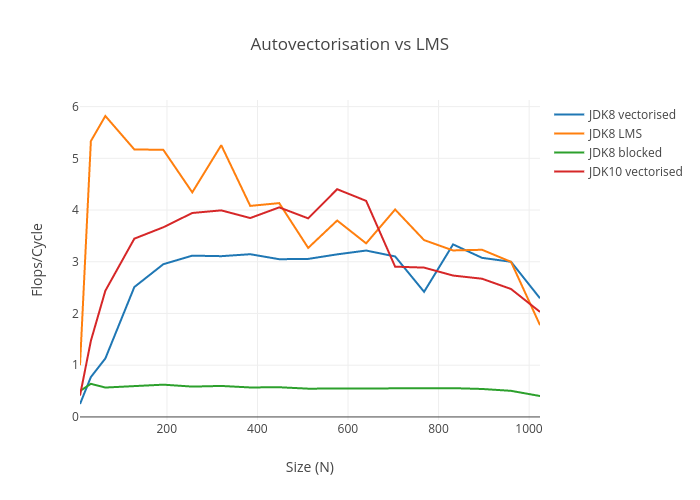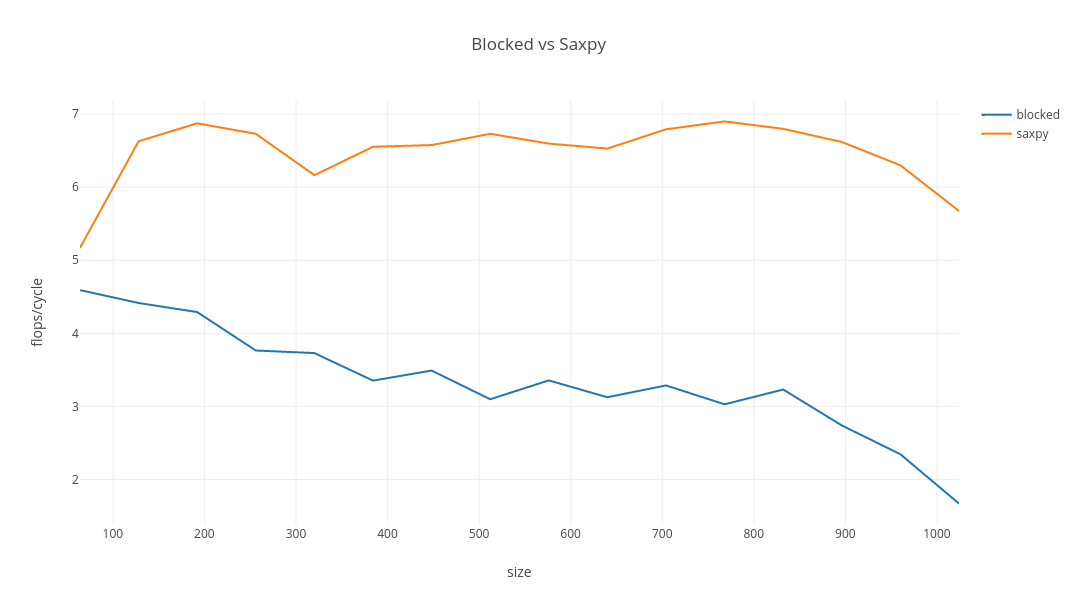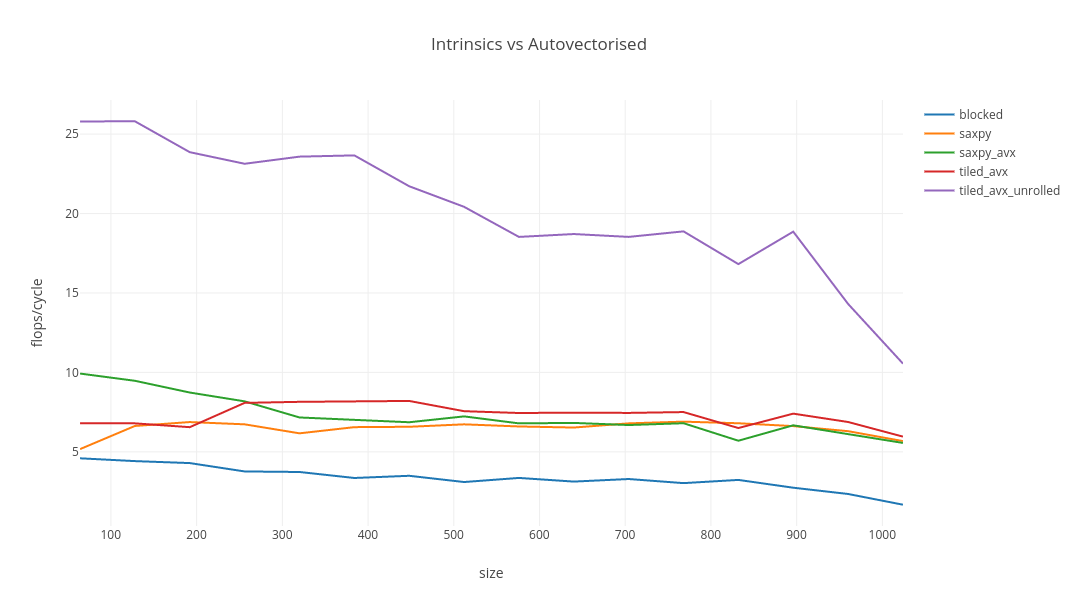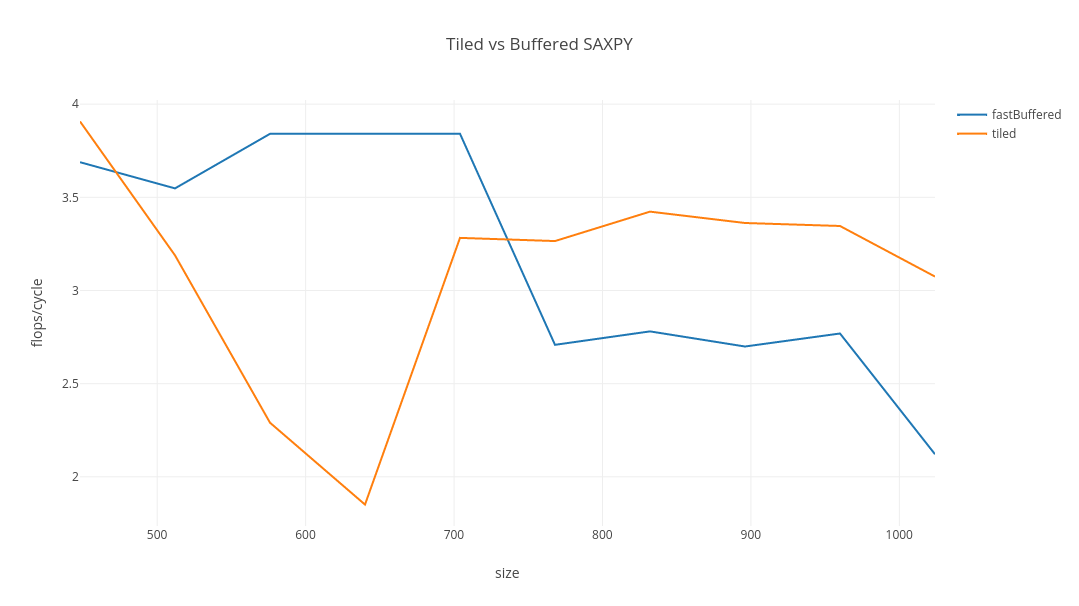In a recent post, I took a look at matrix multiplication in pure Java, to see if it can go faster than reported in SIMD Intrinsics on Managed Language Runtimes. I found faster implementations than the paper’s benchmarks implied was possible. Nevertheless, I found that there were some limitations in Hotspot’s autovectoriser that I didn’t expect to see, even in JDK10. Were these limitations somehow fundamental, or can other compilers do better with essentially the same input?

I took a look at the code generated by GCC’s autovectoriser to see what’s possible in C/C++ without resorting to complicated intrinsics. For a bit of fun, I went over to the dark side to squeeze out some a lot of extra performance, which gave inspiration to a simple vectorised Java implementation which can maintain intensity as matrix size increases.

### Background

The paper reports a 5x improvement in matrix multiplication throughput as a result of using LMS generated intrinsics. Using GCC as LMS’s backend, I easily reproduced very good throughput, but I found two Java implementations better than the paper’s baseline. The best performing Java implementation proposed in the paper was blocked. This post is not about the LMS benchmarks, but this code is this post’s inspiration.

public void blocked(float[] a, float[] b, float[] c, int n) {
int BLOCK_SIZE = 8;
// GOOD: attempts to do as much work in submatrices
// GOOD: tries to avoid bringing data through cache multiple times
for (int kk = 0; kk < n; kk += BLOCK_SIZE) {
for (int jj = 0; jj < n; jj += BLOCK_SIZE) {
for (int i = 0; i < n; i++) {
for (int j = jj; j < jj + BLOCK_SIZE; ++j) {
// BAD: manual unrolling, bypasses optimisations
float sum = c[i * n + j];
for (int k = kk; k < kk + BLOCK_SIZE; ++k) {
// BAD: horizontal sums are inefficient
sum += a[i * n + k] * b[k * n + j];
}
c[i * n + j] = sum;
}
}
}
}
}


I proposed the following implementation for improved cache efficiency and expected it to vectorise automatically.

public void fast(float[] a, float[] b, float[] c, int n) {
// GOOD: 2x faster than "blocked" - why?
int in = 0;
for (int i = 0; i < n; ++i) {
int kn = 0;
for (int k = 0; k < n; ++k) {
float aik = a[in + k];
// MIXED: passes over c[in:in+n] multiple times per k-value, "free" if n is small
// MIXED: reloads b[kn:kn+n] repeatedly for each i, bad if n is large, "free" if n is small
// BAD: doesn't vectorise but should
for (int j = 0; j < n; ++j) {
c[in + j] += aik * b[kn + j]; // sequential writes and reads, cache and vectoriser friendly
}
kn += n;
}
in += n;
}
}


My code actually doesn’t vectorise, even in JDK10, which really surprised me because the inner loop vectorises if the offsets are always zero. In any case, there is a simple hack involving the use of buffers, which unfortunately thrashes the cache, but narrows the field significantly.

  public void fastBuffered(float[] a, float[] b, float[] c, int n) {
float[] bBuffer = new float[n];
float[] cBuffer = new float[n];
int in = 0;
for (int i = 0; i < n; ++i) {
int kn = 0;
for (int k = 0; k < n; ++k) {
float aik = a[in + k];
System.arraycopy(b, kn, bBuffer, 0, n);
saxpy(n, aik, bBuffer, cBuffer);
kn += n;
}
System.arraycopy(cBuffer, 0, c, in, n);
Arrays.fill(cBuffer, 0f);
in += n;
}
}


I left the problem looking like this, with the “JDKX vectorised” lines using the algorithm above with a buffer hack:### GCC Autovectorisation

The Java code is very easy to translate into C/C++. Before looking at performance I want to get an idea of what GCC’s autovectoriser does. I want to see the code generated at GCC optimisation level 3, with unrolled loops, FMA, and AVX2, which can be seen as follows:

g++ -mavx2 -mfma -march=native -funroll-loops -O3 -S mmul.cpp

The generated assembly code can be seen in full context here. Let’s look at the mmul_saxpy routine first:

static void mmul_saxpy(const int n, const float* left, const float* right, float* result) {
int in = 0;
for (int i = 0; i < n; ++i) {
int kn = 0;
for (int k = 0; k < n; ++k) {
float aik = left[in + k];
for (int j = 0; j < n; ++j) {
result[in + j] += aik * right[kn + j];
}
kn += n;
}
in += n;
}
}


This routine uses SIMD instructions, which means in principle any other compiler could do this too. The inner loop has been unrolled, but this is only by virtue of the -funroll-loops flag. C2 does this sort of thing as standard, but only for hot loops. In general you might not want to unroll loops because of the impact on code size, and it’s great that a JIT compiler can decide only to do this when it’s profitable.

.L9:
vmovups	(%rdx,%rax), %ymm4
addl	$8, %r10d vmovaps %ymm4, (%r11,%rax) vmovups 32(%rdx,%rax), %ymm5 vfmadd213ps 32(%rbx,%rax), %ymm3, %ymm5 vmovaps %ymm5, 32(%r11,%rax) vmovups 64(%rdx,%rax), %ymm1 vfmadd213ps 64(%rbx,%rax), %ymm3, %ymm1 vmovaps %ymm1, 64(%r11,%rax) vmovups 96(%rdx,%rax), %ymm2 vfmadd213ps 96(%rbx,%rax), %ymm3, %ymm2 vmovaps %ymm2, 96(%r11,%rax) vmovups 128(%rdx,%rax), %ymm4 vfmadd213ps 128(%rbx,%rax), %ymm3, %ymm4 vmovaps %ymm4, 128(%r11,%rax) vmovups 160(%rdx,%rax), %ymm5 vfmadd213ps 160(%rbx,%rax), %ymm3, %ymm5 vmovaps %ymm5, 160(%r11,%rax) vmovups 192(%rdx,%rax), %ymm1 vfmadd213ps 192(%rbx,%rax), %ymm3, %ymm1 vmovaps %ymm1, 192(%r11,%rax) vmovups 224(%rdx,%rax), %ymm2 vfmadd213ps 224(%rbx,%rax), %ymm3, %ymm2 vmovaps %ymm2, 224(%r11,%rax) addq$256, %rax
cmpl	%r10d, 24(%rsp)
ja	.L9


The mmul_blocked routine is compiled to quite convoluted assembly. It has a huge problem with the expression right[k * n + j], which requires a gather and is almost guaranteed to create 8 cache misses per block for large matrices. Moreover, this inefficiency gets much worse with problem size.

static void mmul_blocked(const int n, const float* left, const float* right, float* result) {
int BLOCK_SIZE = 8;
for (int kk = 0; kk < n; kk += BLOCK_SIZE) {
for (int jj = 0; jj < n; jj += BLOCK_SIZE) {
for (int i = 0; i < n; i++) {
for (int j = jj; j < jj + BLOCK_SIZE; ++j) {
float sum = result[i * n + j];
for (int k = kk; k < kk + BLOCK_SIZE; ++k) {
sum += left[i * n + k] * right[k * n + j]; // second read here requires a gather
}
result[i * n + j] = sum;
}
}
}
}
}


This compiles to assembly with the unrolled vectorised loop below:

.L114:
cmpq	%r10, %r9
setbe	%cl
cmpq	56(%rsp), %r8
setnb	%dl
orl	%ecx, %edx
cmpq	%r14, %r9
setbe	%cl
cmpq	64(%rsp), %r8
setnb	%r15b
orl	%ecx, %r15d
andl	%edx, %r15d
cmpq	%r11, %r9
setbe	%cl
cmpq	48(%rsp), %r8
setnb	%dl
orl	%ecx, %edx
andl	%r15d, %edx
cmpq	%rbx, %r9
setbe	%cl
cmpq	40(%rsp), %r8
setnb	%r15b
orl	%ecx, %r15d
andl	%edx, %r15d
cmpq	%rsi, %r9
setbe	%cl
cmpq	32(%rsp), %r8
setnb	%dl
orl	%ecx, %edx
andl	%r15d, %edx
cmpq	%rdi, %r9
setbe	%cl
cmpq	24(%rsp), %r8
setnb	%r15b
orl	%ecx, %r15d
andl	%edx, %r15d
cmpq	%rbp, %r9
setbe	%cl
cmpq	16(%rsp), %r8
setnb	%dl
orl	%ecx, %edx
andl	%r15d, %edx
cmpq	%r12, %r9
setbe	%cl
cmpq	8(%rsp), %r8
setnb	%r15b
orl	%r15d, %ecx
testb	%cl, %dl
je	.L111
leaq	32(%rax), %rdx
cmpq	%rdx, %r8
setnb	%cl
cmpq	%rax, %r9
setbe	%r15b
orb	%r15b, %cl
je	.L111
vmovups	(%r8), %ymm2
vmovups	%ymm0, (%r8)


### Benchmarks

I implemented a suite of benchmarks to compare the implementations. You can run them, but since they measure throughput and intensity averaged over hundreds of iterations per matrix size, the full run will take several hours.

g++ -mavx2 -mfma -march=native -funroll-loops -O3 mmul.cpp -o mmul.exe && ./mmul.exe > results.csv

The saxpy routine wins, with blocked fading fast after a middling start.name size throughput (ops/s) flops/cycle
blocked 64 22770.2 4.5916
saxpy 64 25638.4 5.16997
blocked 128 2736.9 4.41515
saxpy 128 4108.52 6.62783
blocked 192 788.132 4.29101
saxpy 192 1262.45 6.87346
blocked 256 291.728 3.76492
saxpy 256 521.515 6.73044
blocked 320 147.979 3.72997
saxpy 320 244.528 6.16362
blocked 384 76.986 3.35322
saxpy 384 150.441 6.55264
blocked 448 50.4686 3.4907
saxpy 448 95.0752 6.57594
blocked 512 30.0085 3.09821
saxpy 512 65.1842 6.72991
blocked 576 22.8301 3.35608
saxpy 576 44.871 6.59614
blocked 640 15.5007 3.12571
saxpy 640 32.3709 6.52757
blocked 704 12.2478 3.28726
saxpy 704 25.3047 6.79166
blocked 768 8.69277 3.02899
saxpy 768 19.8011 6.8997
blocked 832 7.29356 3.23122
saxpy 832 15.3437 6.7976
blocked 896 4.95207 2.74011
saxpy 896 11.9611 6.61836
blocked 960 3.4467 2.34571
saxpy 960 9.25535 6.29888
blocked 1024 2.02289 1.67082
saxpy 1024 6.87039 5.67463

With GCC autovectorisation, saxpy performs well, maintaining intensity as size increases, albeit well below the theoretical capacity. It would be nice if similar code could be JIT compiled in Java.

### Intel Intrinsics

To understand the problem space a bit better, I find out how fast matrix multiplication can get without domain expertise by handcrafting an algorithm with intrinsics. My laptop’s Skylake chip (turbo boost and hyperthreading disabled) is capable of 32 SP flops per cycle per core - Java and the LMS implementation previously fell a long way short of that. It was difficult getting beyond 4f/c with Java, and LMS peaked at almost 6f/c before quickly tailing off. GCC autovectorisation achieved and maintained 7f/c.

To start, I’ll take full advantage of the facility to align the matrices on 64 byte intervals, since I have 64B cache lines, though this might just be voodoo. I take the saxpy routine and replace its kernel with intrinsics. Because of the -funroll-loops option, this will get unrolled without effort.

static void mmul_saxpy_avx(const int n, const float* left, const float* right, float* result) {
int in = 0;
for (int i = 0; i < n; ++i) {
int kn = 0;
for (int k = 0; k < n; ++k) {
__m256 aik = _mm256_set1_ps(left[in + k]);
int j = 0;
for (; j < n; j += 8) {
}
for (; j < n; ++j) {
result[in + j] += left[in + k] * right[kn + j];
}
kn += n;
}
in += n;
}
}


This code is actually not a lot faster, if at all, than the basic saxpy above: a lot of aggressive optimisations have already been applied.

### Combining Blocked and SAXPY

What makes blocked so poor is the gather and the cache miss, not the concept of blocking itself. A limiting factor for saxpy performance is that the ratio of loads to floating point operations is too high. With this in mind, I tried combining the blocking idea with saxpy, by implementing saxpy multiplications for smaller sub-matrices. This results in a different algorithm with fewer loads per floating point operation, and the inner two loops are swapped. It avoids the gather and the cache miss in blocked. Because the matrices are in row major format, I make the width of the blocks much larger than the height. Also, different heights and widths make sense depending on the size of the matrix, so I choose them dynamically. The design constraints are to avoid gathers and horizontal reduction.

static void mmul_tiled_avx(const int n, const float *left, const float *right, float *result) {
const int block_width = n >= 256 ? 512 : 256;
const int block_height = n >= 512 ? 8 : n >= 256 ? 16 : 32;
for (int row_offset = 0; row_offset < n; row_offset += block_height) {
for (int column_offset = 0; column_offset < n; column_offset += block_width) {
for (int i = 0; i < n; ++i) {
for (int j = column_offset; j < column_offset + block_width && j < n; j += 8) {
__m256 sum = _mm256_load_ps(result + i * n + j);
for (int k = row_offset; k < row_offset + block_height && k < n; ++k) {
sum = _mm256_fmadd_ps(_mm256_set1_ps(left[i * n + k]), _mm256_load_ps(right + k * n + j), sum);
}
_mm256_store_ps(result + i * n + j, sum);
}
}
}
}
}


You will see in the benchmark results that this routine really doesn’t do very well compared to saxpy. Finally, I unroll it, which is profitable despite setting -funroll-loops because there is slightly more to this than an unroll. This is a sequence of vertical reductions which have no data dependencies.

static void mmul_tiled_avx_unrolled(const int n, const float *left, const float *right, float *result) {
const int block_width = n >= 256 ? 512 : 256;
const int block_height = n >= 512 ? 8 : n >= 256 ? 16 : 32;
for (int column_offset = 0; column_offset < n; column_offset += block_width) {
for (int row_offset = 0; row_offset < n; row_offset += block_height) {
for (int i = 0; i < n; ++i) {
for (int j = column_offset; j < column_offset + block_width && j < n; j += 64) {
__m256 sum1 = _mm256_load_ps(result + i * n + j);
__m256 sum2 = _mm256_load_ps(result + i * n + j + 8);
__m256 sum3 = _mm256_load_ps(result + i * n + j + 16);
__m256 sum4 = _mm256_load_ps(result + i * n + j + 24);
__m256 sum5 = _mm256_load_ps(result + i * n + j + 32);
__m256 sum6 = _mm256_load_ps(result + i * n + j + 40);
__m256 sum7 = _mm256_load_ps(result + i * n + j + 48);
__m256 sum8 = _mm256_load_ps(result + i * n + j + 56);
for (int k = row_offset; k < row_offset + block_height && k < n; ++k) {
__m256 multiplier = _mm256_set1_ps(left[i * n + k]);
sum2 = _mm256_fmadd_ps(multiplier, _mm256_load_ps(right + k * n + j + 8), sum2);
sum3 = _mm256_fmadd_ps(multiplier, _mm256_load_ps(right + k * n + j + 16), sum3);
sum4 = _mm256_fmadd_ps(multiplier, _mm256_load_ps(right + k * n + j + 24), sum4);
sum5 = _mm256_fmadd_ps(multiplier, _mm256_load_ps(right + k * n + j + 32), sum5);
sum6 = _mm256_fmadd_ps(multiplier, _mm256_load_ps(right + k * n + j + 40), sum6);
sum7 = _mm256_fmadd_ps(multiplier, _mm256_load_ps(right + k * n + j + 48), sum7);
sum8 = _mm256_fmadd_ps(multiplier, _mm256_load_ps(right + k * n + j + 56), sum8);
}
_mm256_store_ps(result + i * n + j, sum1);
_mm256_store_ps(result + i * n + j + 8, sum2);
_mm256_store_ps(result + i * n + j + 16, sum3);
_mm256_store_ps(result + i * n + j + 24, sum4);
_mm256_store_ps(result + i * n + j + 32, sum5);
_mm256_store_ps(result + i * n + j + 40, sum6);
_mm256_store_ps(result + i * n + j + 48, sum7);
_mm256_store_ps(result + i * n + j + 56, sum8);
}
}
}
}
}


This final implementation is fast, and is probably as good as I am going to manage, without reading papers. This should be a CPU bound problem because the algorithm is O(n^3) whereas the problem size is O(n^2). But the flops/cycle decreases with problem size in all of these implementations. It’s possible that this could be amelioarated by a better dynamic tiling policy. I’m unlikely to be able to fix that.

It does make a huge difference being able to go very low level - handwritten intrinsics with GCC unlock awesome throughput - but it’s quite hard to actually get to the point where you can beat a good optimising compiler. Mind you, there are harder problems to solve this, and you may well be a domain expert.

The benchmark results summarise this best:name size throughput (ops/s) flops/cycle
saxpy_avx 64 49225.7 9.92632
tiled_avx 64 33680.5 6.79165
tiled_avx_unrolled 64 127936 25.7981
saxpy_avx 128 5871.02 9.47109
tiled_avx 128 4210.07 6.79166
tiled_avx_unrolled 128 15997.6 25.8072
saxpy_avx 192 1603.84 8.73214
tiled_avx 192 1203.33 6.55159
tiled_avx_unrolled 192 4383.09 23.8638
saxpy_avx 256 633.595 8.17689
tiled_avx 256 626.157 8.0809
tiled_avx_unrolled 256 1792.52 23.1335
saxpy_avx 320 284.161 7.1626
tiled_avx 320 323.197 8.14656
tiled_avx_unrolled 320 935.571 23.5822
saxpy_avx 384 161.517 7.03508
tiled_avx 384 188.215 8.19794
tiled_avx_unrolled 384 543.235 23.6613
saxpy_avx 448 99.1987 6.86115
tiled_avx 448 118.588 8.2022
tiled_avx_unrolled 448 314 21.718
saxpy_avx 512 70.0296 7.23017
tiled_avx 512 73.2019 7.55769
tiled_avx_unrolled 512 197.815 20.4233
saxpy_avx 576 46.1944 6.79068
tiled_avx 576 50.6315 7.44294
tiled_avx_unrolled 576 126.045 18.5289
saxpy_avx 640 33.8209 6.81996
tiled_avx 640 37.0288 7.46682
tiled_avx_unrolled 640 92.784 18.7098
saxpy_avx 704 24.9096 6.68561
tiled_avx 704 27.7543 7.44912
tiled_avx_unrolled 704 69.0399 18.53
saxpy_avx 768 19.5158 6.80027
tiled_avx 768 21.532 7.50282
tiled_avx_unrolled 768 54.1763 18.8777
saxpy_avx 832 12.8635 5.69882
tiled_avx 832 14.6666 6.49766
tiled_avx_unrolled 832 37.9592 16.8168
saxpy_avx 896 12.0526 6.66899
tiled_avx 896 13.3799 7.40346
tiled_avx_unrolled 896 34.0838 18.8595
saxpy_avx 960 8.97193 6.10599
tiled_avx 960 10.1052 6.87725
tiled_avx_unrolled 960 21.0263 14.3098
saxpy_avx 1024 6.73081 5.55935
tiled_avx 1024 7.21214 5.9569
tiled_avx_unrolled 1024 12.7768 10.5531

### Can we do better in Java?

Writing genuinely fast code gives an indication of how little of the processor Java actually utilises, but is it possible to bring this knowledge over to Java? The saxpy based implementations in my previous post performed well for small to medium sized matrices. Once the matrices grow, however, they become too big to be allowed to pass through cache multiple times: we need hot, small cached data to be replenished from the larger matrix. Ideally we wouldn’t need to make any copies, but it seems that the autovectoriser doesn’t like offsets: System.arraycopy is a reasonably fast compromise. The basic sequential read pattern is validated: even native code requiring a gather does not perform well for this problem. The best effort C++ code translates almost verbatim into this Java code, which is quite fast for large matrices.

public void tiled(float[] a, float[] b, float[] c, int n) {
final int bufferSize = 512;
final int width = Math.min(n, bufferSize);
final int height = Math.min(n, n >= 512 ? 8 : n >= 256 ? 16 : 32);
float[] sum = new float[bufferSize];
float[] vector = new float[bufferSize];
for (int rowOffset = 0; rowOffset < n; rowOffset += height) {
for (int columnOffset = 0; columnOffset < n; columnOffset += width) {
for (int i = 0; i < n; ++i) {
for (int j = columnOffset; j < columnOffset + width && j < n; j += width) {
int stride = Math.min(n - columnOffset, bufferSize);
// copy to give autovectorisation a hint
System.arraycopy(c, i * n + j, sum, 0, stride);
for (int k = rowOffset; k < rowOffset + height && k < n; ++k) {
float multiplier = a[i * n + k];
System.arraycopy(b, k * n  + j, vector, 0, stride);
for (int l = 0; l < stride; ++l) {
sum[l] = Math.fma(multiplier, vector[l], sum[l]);
}
}
System.arraycopy(sum, 0, c, i * n + j, stride);
}
}
}
}
}


Benchmarking it using the same harness used in the previous post, the performance is ~10% higher for large arrays than my previous best effort. Still, the reality is that this is too slow to be useful. If you need to do linear algebra, use C/C++ for the time being!Benchmark Mode Threads Samples Score Score Error (99.9%) Unit Param: size flops/cycle
fastBuffered thrpt 1 10 53.331195 0.270526 ops/s 448 3.688688696
fastBuffered thrpt 1 10 34.365765 0.16641 ops/s 512 3.548072999
fastBuffered thrpt 1 10 26.128264 0.239719 ops/s 576 3.840914622
fastBuffered thrpt 1 10 19.044509 0.139197 ops/s 640 3.84031059
fastBuffered thrpt 1 10 14.312154 1.045093 ops/s 704 3.841312378
fastBuffered thrpt 1 10 7.772745 0.074598 ops/s 768 2.708411991
fastBuffered thrpt 1 10 6.276182 0.067338 ops/s 832 2.780495238
fastBuffered thrpt 1 10 4.8784 0.067368 ops/s 896 2.699343067
fastBuffered thrpt 1 10 4.068907 0.038677 ops/s 960 2.769160387
fastBuffered thrpt 1 10 2.568101 0.108612 ops/s 1024 2.121136502
tiled thrpt 1 10 56.495366 0.584872 ops/s 448 3.907540754
tiled thrpt 1 10 30.884954 3.221017 ops/s 512 3.188698735
tiled thrpt 1 10 15.580581 0.412654 ops/s 576 2.290381075
tiled thrpt 1 10 9.178969 0.841178 ops/s 640 1.850932038
tiled thrpt 1 10 12.229763 0.350233 ops/s 704 3.282408783
tiled thrpt 1 10 9.371032 0.330742 ops/s 768 3.265334889
tiled thrpt 1 10 7.727068 0.277969 ops/s 832 3.423271628
tiled thrpt 1 10 6.076451 0.30305 ops/s 896 3.362255222
tiled thrpt 1 10 4.916811 0.2823 ops/s 960 3.346215151
tiled thrpt 1 10 3.722623 0.26486 ops/s 1024 3.074720008